# Seating Arrangement

'Seating Arrangement' is a sequential arrangement of objects/persons on the basis of predefined conditions.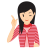Few Important things to Remember

In this section, we deal with the questions based on setting arrangement of persons or objects. In this type of questions, some conditions are given, on the basis of which candidates are required to arrange objects/person, either in a row or in a circular order or in any other geometrical shape. While making arrangements, it should be noted that all the conditions given are complied with.

Types of seating arrangements:

1. Circular Seating Arrangement
2. Row or Column Seating Arrangement
3. Square Seating Arrangement

In order to solve seating arrangement questions, first of all diagram should be made. By doing so questions are easily and quickly solved.

## Types of Questions

There are three types of questions, which are generally asked in various competitive examinations.

Type #1: Arrangement in Linear Pattern

Here, we deal with questions, where a proper arrangement is required to be done in a linear format along a straight line.

Before solving this type of questions, it is necessary to know the given facts.

• When it is not given in the question that in which direction the person(s) is facing, then we assume it facing the North direction.

• When A, B, C and D are facing in South direction and P, Q, R and S are facing in North direction in a line, the positions to their right and left will be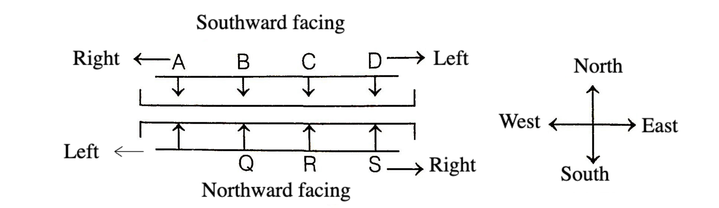• When A, B are in one line and P, Q in other, then diagonally opposite directions will beA is diagonally opposite to Q and B is diagonally opposite to P.

Example 1: P, Q, R, S and T are sitting in a line facing West. P and Q are sitting together. R is sitting at south end and S is sitting at North end. T is neighbour of Q and R. Who is sitting the middle?

(a) P          (b) Q         (c) R         (d) S

Solution:  (b) According to the given information, the sitting arrangement is a given below.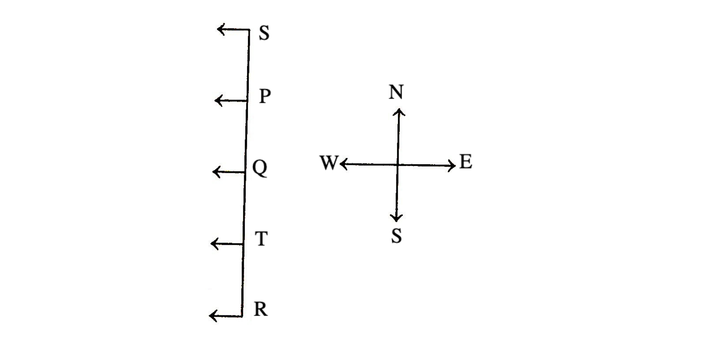Clearly, from the above diagram, Q is setting in the middle.

Directions (Example Nos. 2-5) Study the following information carefully and answer the questions given below.

Six friends A, B, C, D, E and F are sitting in a row facing towards North. C is sitting between A and E. D is not at the extreme end. B is sitting at immediate right of E. F is not at the right end but D is sitting at 3rd left of E.

Example 2: How many persons are there to the right of D?

(a) One      (b) Two      (c) Three      (d) Four      (e) None of these

Example 3: Which of the following is setting to the left of D?

(a) F          (b) C           (c) E               (d) A          (e) None of these

Example 4: Who is at the immediate left of C?

(a) A          (b) E           (c) Either E or A

(d) Cannot be determined

(e) None of these

Example 5: Who is at the right end?

(a) A              (b) B                  (c) E

(d) Cannot be determined

(e) None of these

Solution:  (Example Nos. 2-5) According to the given information, the sitting arrangement of six friends is as given below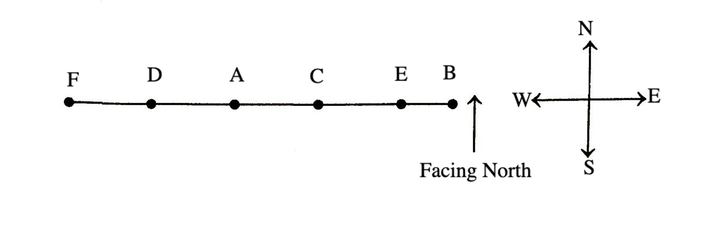2. (d) It is clear from the diagram that there are four persons to the right of D ----- A, C, E and B.

3. (a) It is clear from the diagram that F is sitting to the left of D.

4. (a) It is clear from the diagram that A is at the immediate left of C.

5. (b) It is clear from the diagram that B is at the right end.

Example 6: Six persons A, B, C, D, E and F sit in two rows of three persons each. If E is not at any end of rows, D is second to the left of F, C is the neighbour of E and is sitting diagonally opposite to D and B is the neighbour of F, then who will sit opposite B?

(a) A         (b) E        (c) C         (d) D

Solution:  (b) According to the given question, the arrangements are as follows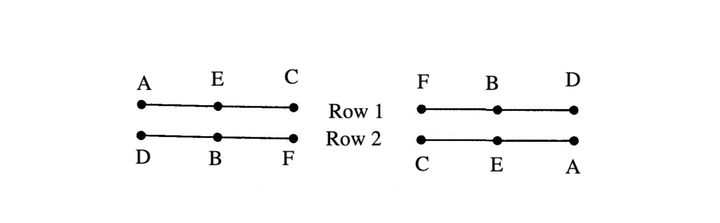Hence, E is sitting opposite to B.

Note : Linear arrangement also includes floor arrangement in which people are arranged as per their floor numbers from top to bottom. The lowest floor is usually numbered 1.

Directions (Example Nos. 7-9) Study the following information carefully and answer the questions given below.

There are eight persons namely S, T, U, V, W, X, Y and Z lives on eight different floors from one to eight. Ground floor is number one and one above that is number two and so on till the topmost floor is number eight. X lives on odd number floor but does not live on 3rd floor. Z lives immediate below X. More than two person lives between Z and Y. There are six person lives between S and Y. V lives immediate above W, but live below T. U does not live above X. W does not live immediate above Y.

Example 7: Who lives on floor number five?

(a) U         (b) S         (c) Z          (d) T          (e) None of these

Example 8: How many persons live between W and X?

(a) One     (b) Three     (c) Five      (d) Two      (e) None of these

Example 9: Who lives immediately above V?

(a) Z          (b) T             (c) Y           (d) W          (e) None of these

Solution:  (Example Nos. 7-9) According to the given information, the arrangement is as follows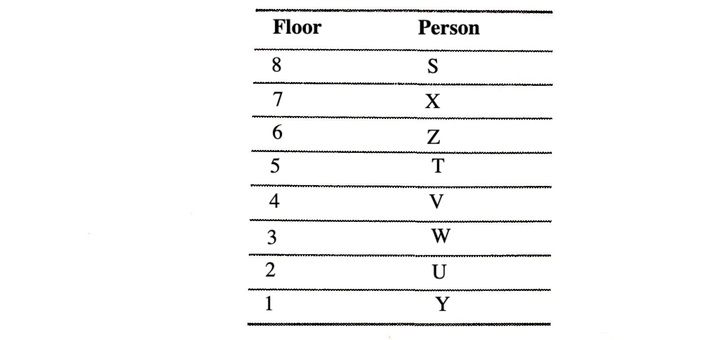7. (d) T lives on floor number five.

8. (b) Three person live between W and X.

9. (b) T lives immediately above V.

Type #2: Circular Arrangement

In circular arrangement, persons/objects are arranged around a circle facing towards or outside the centre on the basis of information provided.

Facing Towards the Centre

Suppose A, B, C and D are four persons facing towards the centre, then their right and left sides are shown by the arrows in the given diagram. B is on the right side of A and D is on the left side of A.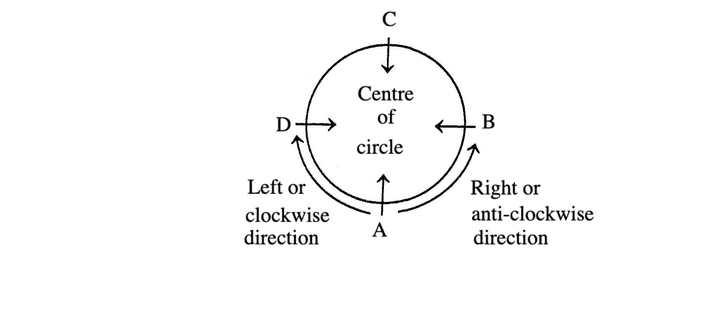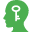Memory Add-ons

Facing Centre

• Towards Left – Clockwise Rotation.

• Towards Right – Anticlockwise Rotation.

Facing Outside the Centre

Suppose there are four persons P, Q, R and S. All the persons are facing outside the centre, then their left and right sides are shown in the diagram by arrows. Q is on the right of P and S is on the left of P.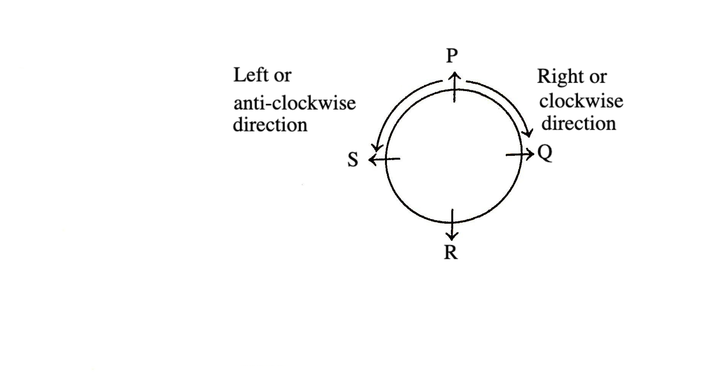Memory Add-ons

Facing Outwards

• Towards Left – Anticlockwise Rotation.

• Towards Right – Clockwise Rotation.

MIND IT !

When it is not given in the question that in which direction the persons are facing then we assume it facing the centre.

Example 1: Five students are standing in a circle. Abhinav is between Alok and Ankur. Apurva is on the left of Abhishek. Alok is on the left of Apurva. Who is sitting next to Abhinav on his right?

(a) Apurva                 (b) Ankur

(c) Abhishek             (d) Alok

Solution:  (d) We arrange the following students in a circle based on the information given in the question.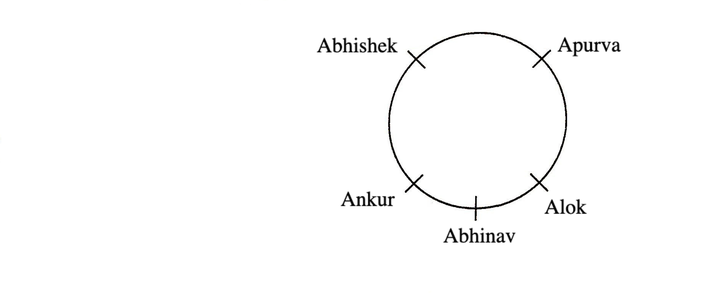Clearly, Alok is sitting next of Abhinav on his right.

Directions (Example Nos. 2-4) Study the following information carefully and answer the questions given below.

Eight persons I, J, K, L, M, N, O and P are sitting around a circular table but not necessarily in the same order. Three of them are facing outwards while five are facing towards the centre.

M is third to the right of K. N is sitting third to the left of M. Three persons are sitting between N and J. O is sitting third to the right of N, who is not facing the centre. L is sitting third to the right of I, who is not facing the centre.

Example 2: Who sits between N and I?

(a) J          (b) K        (c) M        (d) O
(e) None of these

Example 3: Who among the following is second to the right of J?

(a) L        (b) K           (c) M       (d) N
(e) None of these

Example 4: Which of the following statements is / are true with respect to P?

(a) P is opposite of O

(b) P is fourth to the right of O

(c) P is fourth to the left of O

(d) All are true

(e) None of the above

Solution:  (Example Nos. 2-4) According to the given information, the final arrangement of eight persons around a circular table is shown below.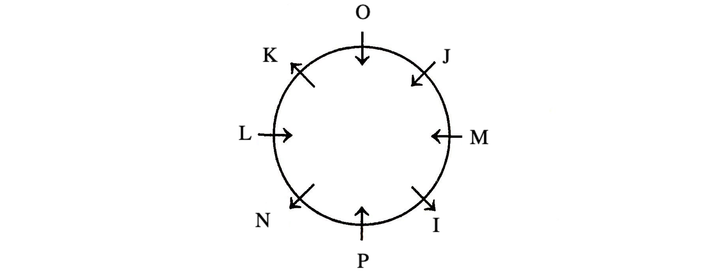2. (e) P is sitting between N and I.

3. (b) K is sitting second to right of J.

4. (d) All statements are true with respect to P.

Type #3: Arrangement in Other Geometrical Shapes

In this type, questions are asked to arrange the person in square, rectangle, pentagon, hexagon or any other geometrical shape.

1. Square/Rectangular Arrangement

In this type of arrangement, objects/persons are placed around a rectangular or a square shaped table facing either at the centre or the direction opposite to centre. The left and right of each person in both the cases can be understood with the help of following diagrams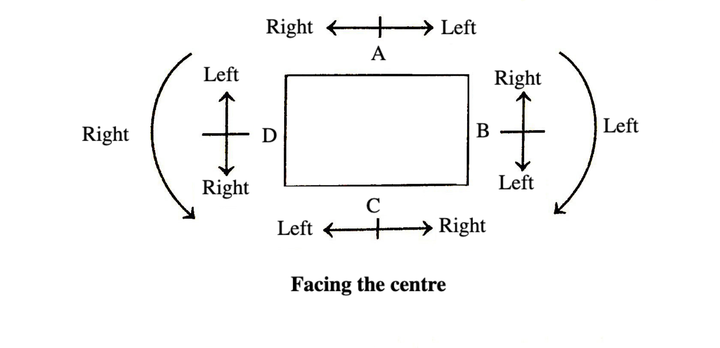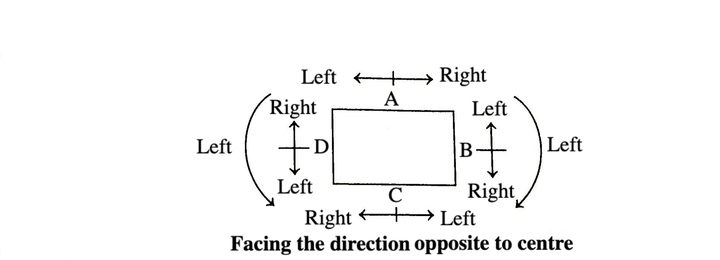2. Pentagonal Arrangement

In this arrangement, persons/objects are placed around a pentagonal plane facing either at the centre or the direction opposite to the centre. The left and right of each persons in both the cases can be understood with the help of the following diagrams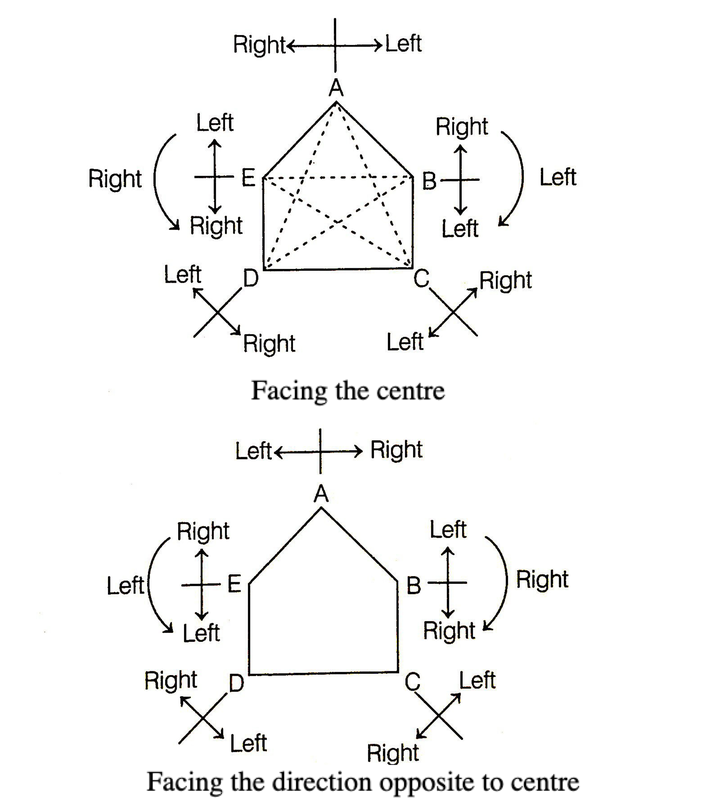3. Hexagonal Arrangement

In this arrangement, objects/persons are placed around a hexagonal plane facing either at the centre or the direction opposite to centre. The left and right of each person/object in both the cases can be understood with the help of following diagrams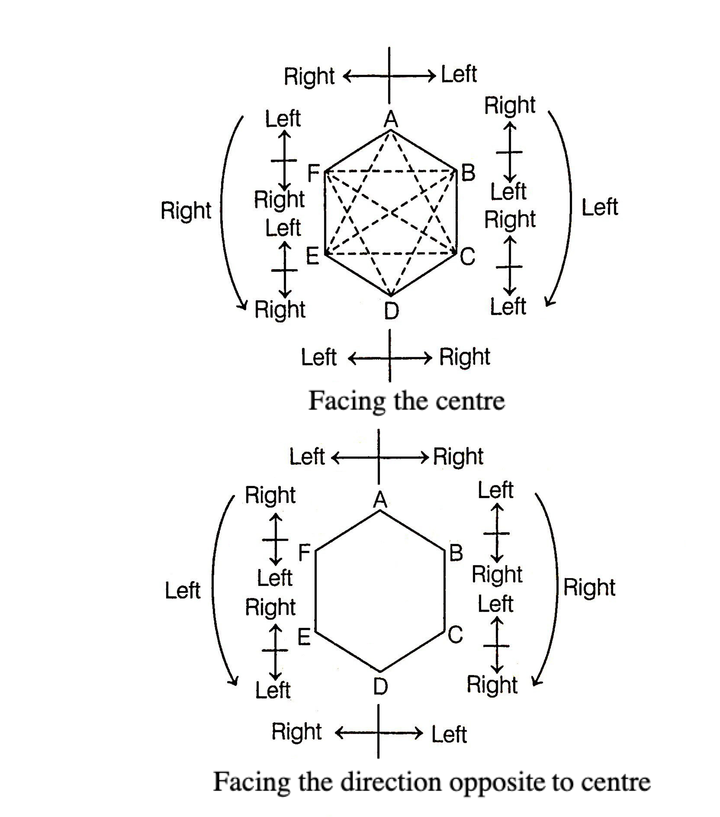Example 1: A, B, C and D are playing cards on square table.

A and C and B and D are partners. D is to the right of C. The face of C is towards West. Find the direction that D is facing,

(a) West         (b) East        (c) South         (d) North

Solution:  (c) Arrangement according to the question is a follows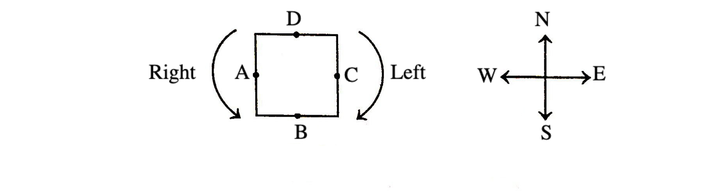Clearly, D is facing South.

Directions (Example Nos. 2-4) Study the following information carefully and answer the questions given below.

Eight friends P, Q, R, S, T, V, W and Y are sitting around a square table in such a way that four of them sit at four corners of the square while four sit in the middle of each of the four sides. The ones who sit at the four corners face the centre while those who sit in the middle of the sides face outside.

P who faces the centre sits third to the right of V. T who faces the centre, is not an immediate neighbour of V. Only one person sit between V and W. S sits second of right of Q. Q faces the centre. R is not an immediate neighbour of P.

Example 2: Who sit second to the left of Q?

(a) V         (b) P         (c) T          (d) Y          (e) Cannot be determined

Example 3: What is the person of T with respect of V?

(a) Fourth to the left              (b) Second to the left

(c) Third to the left                 (d) Third to the right

(e)Second to the right

Example 4: Four of the following five are alike in certain way and so form a group. Which is the one that does not belong to that group?

(a) R         (b) W         (c) V          (d) S          (e) Y

Solution:  (Example Nos. 2-4) On the basis of given information, the seating arrangement is as given below2. (b) Q faces centre and P is second to the left of Q.

3. (c) T is third to the left of V

4. (d) R, W, V and Y are sitting in the middle of the sides of the table. S is sitting at the corner.

Example 5: A, B, C, D, E, F, G and H are eight girls who are sitting around an octagonal enclosure facing the centre. B is sitting between G and D, H is third to the left of B and second to the right of A, C is sitting between A and G, B and E are not sitting opposite to each other .
Who is sitting third to the left of D?

(a) H          (b) G        (c) F        (d) E

Solution: (c) Following sitting arrangement can be drawn from the given instructions as given below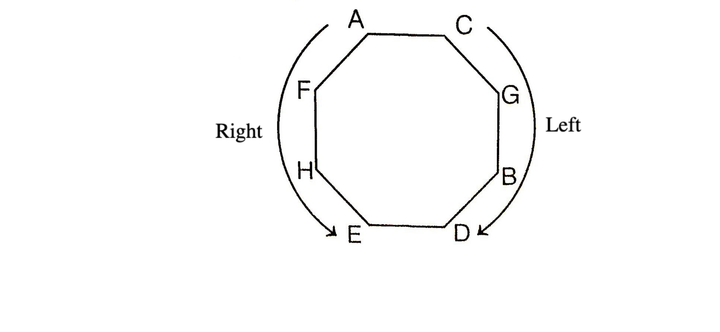Clearly, F is sitting third to the left of D.

LearnFrenzy provides you lots of fully solved Seating Arrangement Questions and Answers with explanation.